Question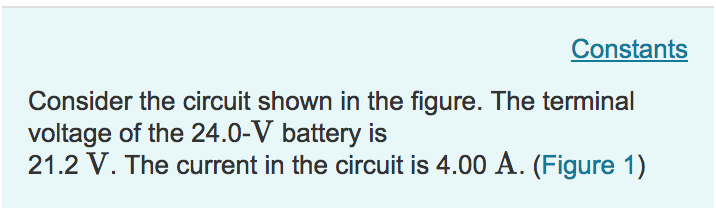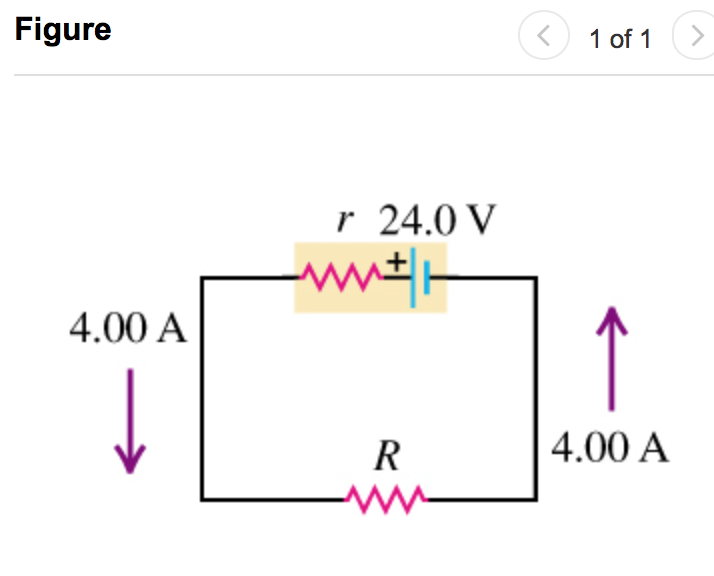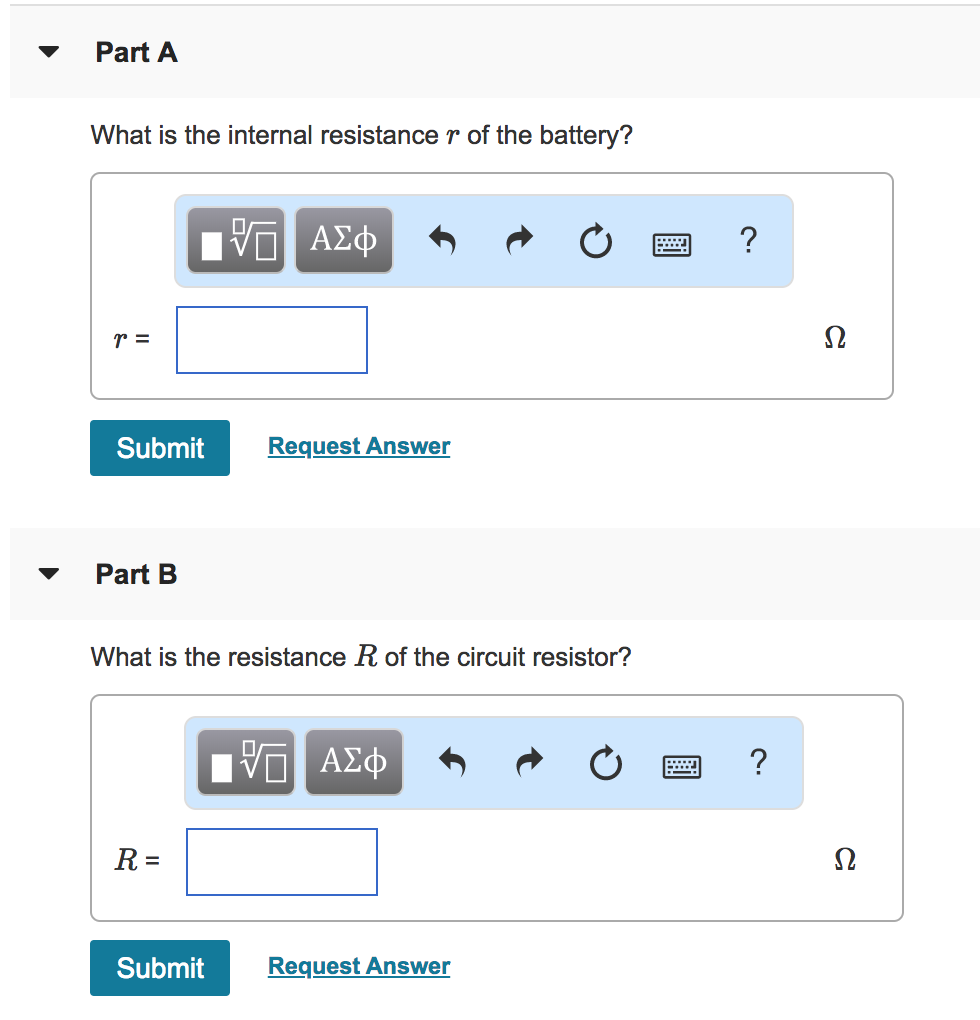Consider the circuit shown in the figure. The terminal voltage of the 24.0-V battery is 21.2 V. The current in the circuit is 4.00 A. (Figure 1)

Part A

What is the internal resistance $$r$$ of the battery?

Part B

What is the resistance $$R$$ of the circuit resistor?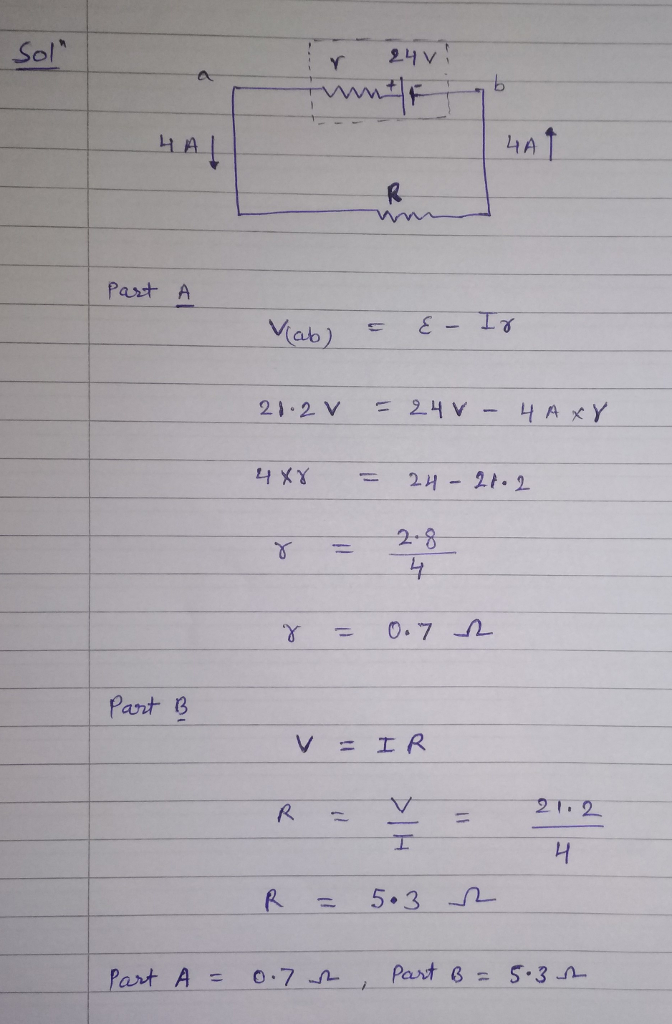#### Earn Coins

Coins can be redeemed for fabulous gifts.

Similar Homework Help Questions
• ### Consider the circuit shown in the figure below. The terminal voltage of the 24.0 V battery...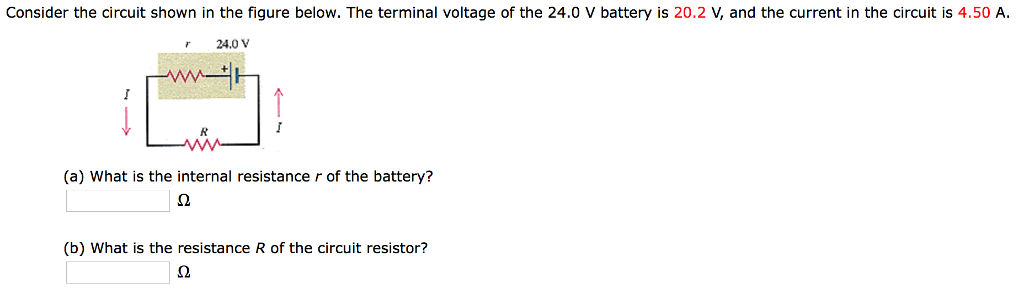Consider the circuit shown in the figure below. The terminal voltage of the 24.0 V battery is 20.2 V, and the current in the circuit is 4.50 A. 24.0V (a) What is the internal resistance r of the battery? (b) What is the resistance R of the circuit resistor?

• ### Consider the circuit shown below. The terminal voltage of the battery is V - 20.00 V...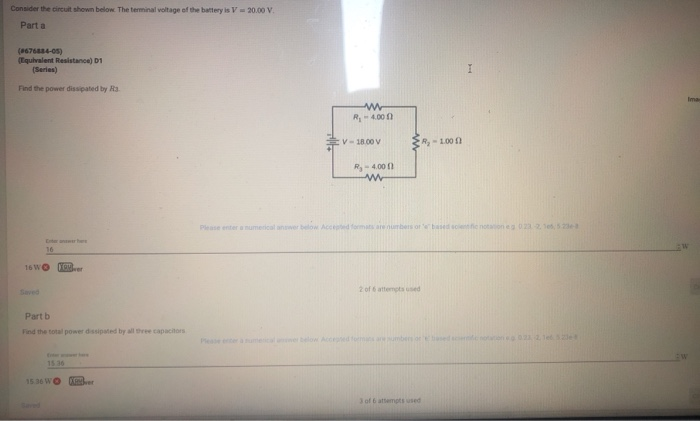Consider the circuit shown below. The terminal voltage of the battery is V - 20.00 V Parta (676684-05) (Equivalent Resistance) D1 Series) Find the power dissipated by a R-400 VIROV R-1000 R - 4.000 M 16W or Part b Find the total power dissipated by tree capacito 1536WO her 3 of 6 attempt used

• ### Consider the electric circuit shown in the figure. Assume that the voltage of the battery is...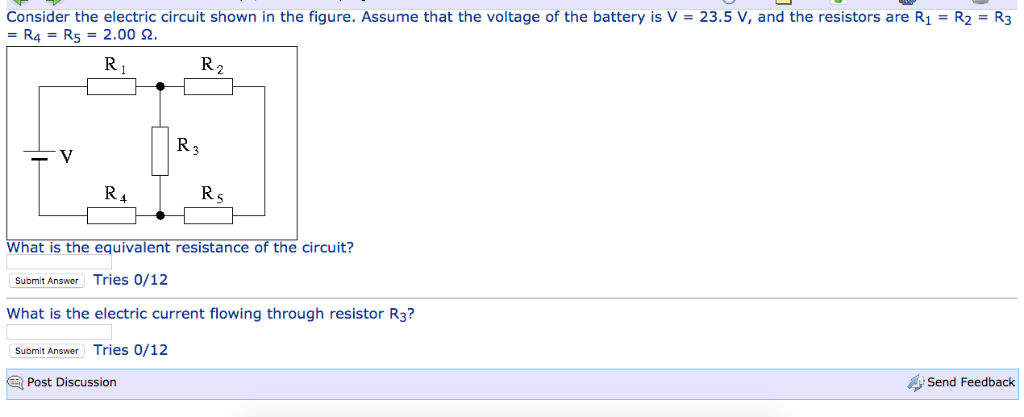Consider the electric circuit shown in the figure. Assume that the voltage of the battery is V = 23.5 V, and the resistors are R1 = R2 = R3 = R4 = R5 = 2.00 Ω. What is the equivalent resistance of the circuit? What is the electric current flowing through resistor R3? Consider the electri = R4 = R5 = 2.00 2 circuit shown in the figure. Assume that the voltage of the battery is V = 23.5 V,...

• ### In the circuit below the voltage supplied by the battery is 24.0 V and the resistance...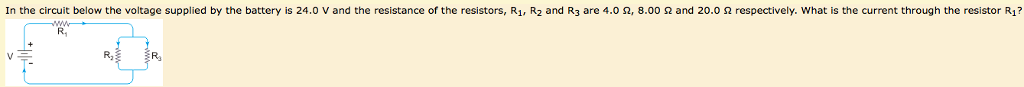In the circuit below the voltage supplied by the battery is 24.0 V and the resistance of the resistors, R1, R2 and R3 are 4.0 Ω, 8.00 Ω and 20.0 Ω respectively, what is the current through the resistor R1? R, R2 Ra What is the current through the resistor R2? What is the current through the resistor R3?

• ### Four resistors are connected to a battery with a terminal voltage of 12 V, as shown...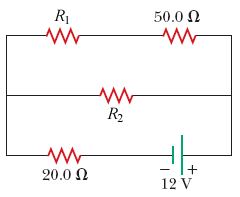Four resistors are connected to a battery with a terminal voltage of 12 V, as shown in the figure below. (Assume R1 = 31.0 Ω and R2 = 70.0 Ω.) (a) How would you reduce the circuit to an equivalent single resistor connected to the battery? Use this procedure to find the equivalent resistance of the circuit. Ω (b) Find the current delivered by the battery to this equivalent resistance. A (c) Determine the power delivered by the battery. W...

• ### Consider the circuit shown in the figure below. (Assume R = 10.5.2, R2 = 2.852, and...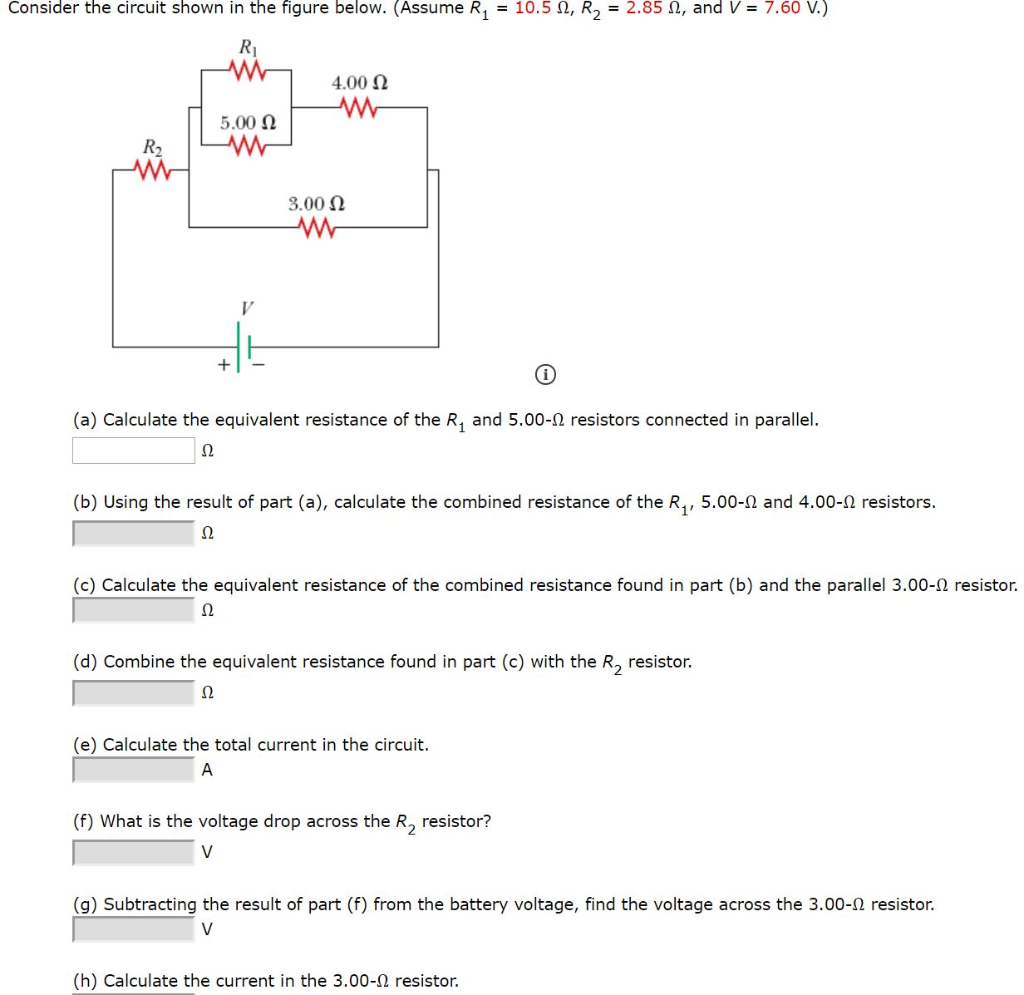Consider the circuit shown in the figure below. (Assume R = 10.5.2, R2 = 2.852, and V = 7.60 V.) R 4.00 12 5.00 12 3.00 12 w (a) Calculate the equivalent resistance of the R. and 5.00-12 resistors connected in parallel. (b) Using the result of part (a), calculate the combined resistance of the Ry, 5.00-12 and 4.00-12 resistors. (c) Calculate the equivalent resistance of the combined resistance found in part (b) and the parallel 3.00-12 resistor. (d) Combine...

• ### Four resistors are connected to a battery with a terminal voltage of 12 V, as shown in the...

Four resistorsare connected to a battery with a terminal voltage of 12 V, as shown in the figure below. Use the following variables as necessary: R1 = 34.0 Ω and R2 = 85.0Ω.(a) How would you reduce the circuit to an equivalent single resistor connected to the battery? Use this procedure to find the equivalent resistance of thecircuit.________ Ω(b) Find the current delivered by the battery to this equivalent resistance.________ A(c) Determine the power delivered by the battery.______ W(d) Determine...

• ### please answer! Part A Terminal Voltage and Internal Resistance: A battery has an emf 8 -...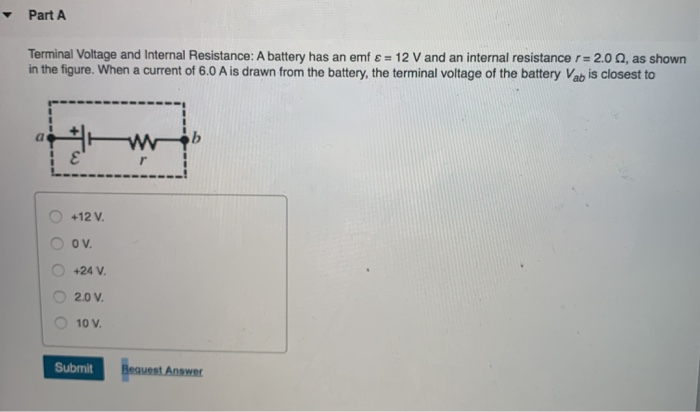please answer! Part A Terminal Voltage and Internal Resistance: A battery has an emf 8 - 12 V and an internal resistance r = 2.0, as shown in the figure. When a current of 6.0 A is drawn from the battery, the terminal voltage of the battery Vab is closest to a b ε' r +12 V. OV. 24 V 2.0 V 10 V. Submit Bequest Answer

• ### A battery has an emf of 15.0 V. The terminal voltage of the battery is 13.0...

A battery has an emf of 15.0 V. The terminal voltage of the battery is 13.0 V when it is delivering 16.0 W of power to an external load resistor R. (a) What is the value of R? ? (b) What is the internal resistance of the battery? ?

• ### Four resistors are connected to a battery with a terminal voltage of 12 v, as shown...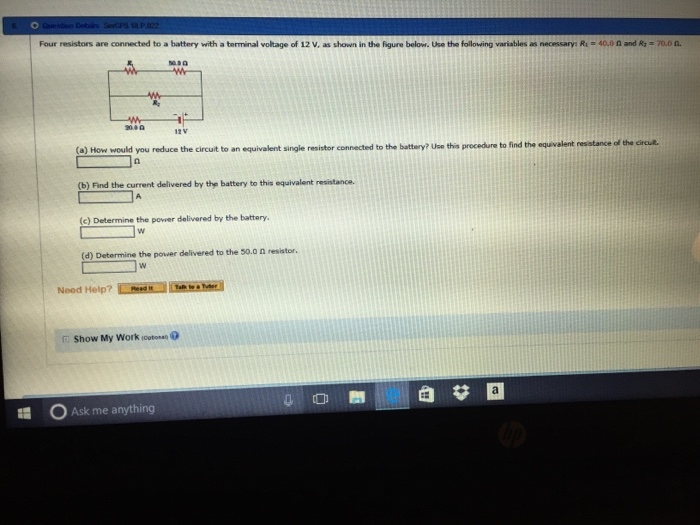Four resistors are connected to a battery with a terminal voltage of 12 v, as shown in the figure below. Use the following variables as neoessaryn Ru 40.0 n and Ra *70.0 in. (a) How would you reduce the circuit to an equivalent single resistor connected to the battery? Uee this to find the equvalent resatance ofthe ercuz. procedure (b) Find the current delivered by the battery to this equivalent resistance. (c) Determine the power delivered by the battery. (d)...